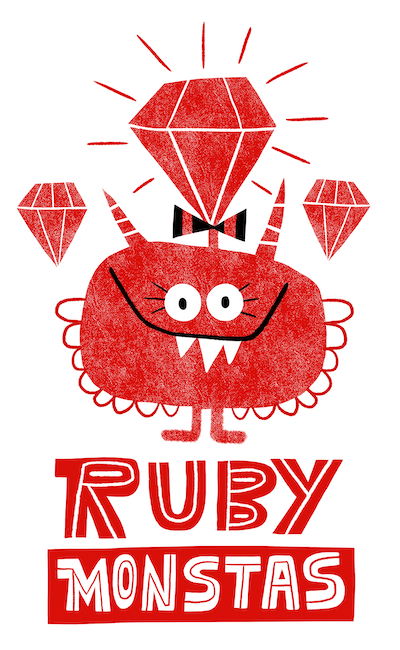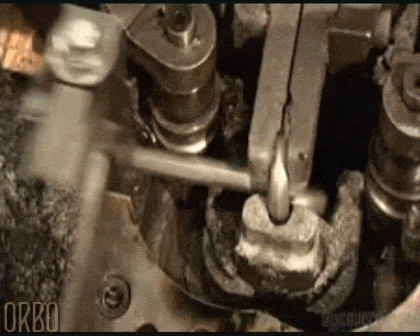# Ruby Monstas

Loops## What is a loop

A loop repeats code until we tell it to stop## A simple loop

We can declare a loop with loop do ... end

``````counter = 0

loop do
puts "#{counter} seconds since the loop started"
sleep(1) # waits for 1 second
counter = counter + 1
end
``````
```0 seconds since the loop started
1 seconds since the loop started
2 seconds since the loop started
3 seconds since the loop started
...```

Problem: This runs forever

## Stopping the loop

We use the keyword break to stop a loop

``````counter = 0

loop do
puts "#{counter} seconds since the loop started"
sleep(1)
counter = counter + 1

if counter == 2
break # "breaks" the loop
end
end

puts "Now we're here..."
``````
```0 seconds since the loop started
1 seconds since the loop started
Now we're here...
```

## Main rule

Every loop should have a break!

...else we have an infinite loop!

## Summary

``````loop do
# the lines between "loop do" and "end"
# get repeated until we
# break it

if condition
break
end         # this "end" belongs to the if-statement
end           # this "end" belongs to loop do
``````